## Projects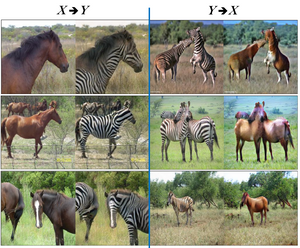ENG EC 500: Deep Learning

Image-To-Image Translation using CycleGAN, November 2018

Implemented GANs and CycleGAN for the task of image translation on multiple paired and unpaired datasets.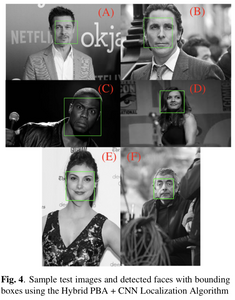ENG EC 520: Digital Image Processing

Face Localization Using Probabilistic Bisection Search and Convolutional Neural Networks, April 2018

Developed an efficient object localization framework using the Probabilistic Bisection Algorithm and a Convolutional Neural Network. Tested the developed method on an image face localization task and successfully located faces.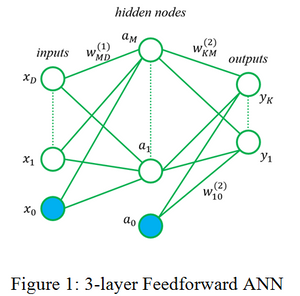ENG EC 503: Learning From Data
Image Classification using Artificial Neural Networks, April 2017

Implemented SVM, Naive Bayes, Logistic Regression, kNN, and Feedforward ANN in MATLAB and applied these methods to an indoor/outdoor image dataset and a 15-scene dataset for image classification.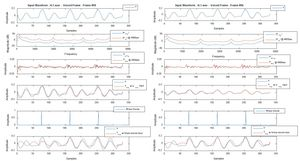ENG EC 519:  Speech Processing
Project 3: Speech Synthesizer – Quantized Binary Source, CELP, May 2016

Created MATLAB code that improved the binary source system from Project 2 and utilized quantization of parameters.  A Coded Excitation Linear Prediction (CELP) system was also implemented using bit allocation schemes.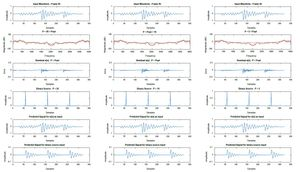ENG EC 519:  Speech Processing
Project 2: Linear Prediction – Analysis and Synthesis, Apr. 2016

Developed a program that carries out Linear Predictive speech analysis and synthesis based on the speech production model.  A digital filter was used to model the vocal tract system function for speech synthesis and the necessary gain and filter coefficient parameters were estimated through Linear Predictive analysis techniques without quantization.  With the vocal tract model, certain inputs for voiced and unvoiced speech were used, namely an impulse train and random noise, respectively, to generate synthesized speech.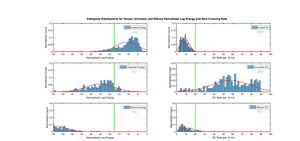ENG EC 519:  Speech Processing
Project 1:  Speech Signal Analyzer, Mar. 2016

Designed MATLAB code that implements 3 algorithms for any speech file: 1) start/end detection, 2) voiced/unvoiced/silence region detection, and 3) pitch estimation.  The program forms a pitch contour and partitions speech signal into its different regions.  These methods are then used for Projects 2 and 3.ENG EC 327:  Introduction to Software Engineering
Password Generator, Mar. 2016 – Apr. 2016

Designed a C++ GUI using Qt that allows users to generate strong passwords based on sets of requirements and store them for future use.  Learned about creating desktop applications using C++.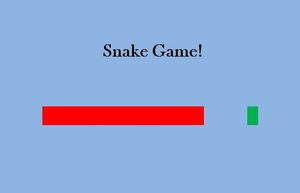ENG EC 311:  Introduction to Logic Design
Snake Game in Verilog, Dec. 2015

Programmed the Snake game in Verilog using an FPGA development board.  The board was used for moving the snake, and the game was displayed on a VGA screen.  A 7-Segment LED display was used to display the user’s current score. Learned about using Verilog on hardware.

ENG EK 132:  Introduction to Engineering, Electronic Control of Robots
iRobot Create Maze Navigation, Apr. 2015

Programmed an iRobot Create to navigate a maze using the C language to navigate a maze.  Learned about robotics and the C programming language.

ENG EK 210:  Introduction to Engineering Design
Feedback Control Device for a Laser Scanning Microscope, Feb. 2015 – Apr. 2015

The goal of the project was to create a feedback control device for a laser scanning microscope that would always keep the sensed signal at a receiving photodetector at a fixed value. A PID controller algorithm was implemented using an Arduino microcontroller to carry out decision processes while a closed loop was filled in with a circuit that mainly consisted of an LED and a photodiode. The LED and photodiode were used to simulate the laser scanning microscope environment.  The adjacent video shows the final apparatus where the desired output voltage of the photodiode, or reference value, is 1 volt.  The LED will change its intensity in order to maintain a constant 1 volt output from the photodiode.  At first, an opaque paper is put between the LED and photodiode, so the photodiode’s output voltage drops and the LED maximizes its brightness to get the photodiode’s output to be 1 volt; this cannot happen since light can’t penetrate the dark paper.  If the dark paper is replaced by a clear plastic, the LED lights up a little and succeeds in maintaining a constant 1 volt output from the photodiode.  From this project, I learned about PID controllers, algorithms, microcontrollers, and engineering design.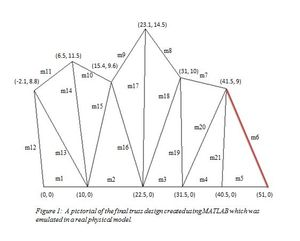ENG EK 301:  Engineering Mechanics – Statics
Truss Design Project, Feb. 2015 – Apr. 2015

Designed and built a truss made of straws to withstand an applied load at a specified joint.  Created a MATLAB program to analyze a given truss design by deriving each member’s buckling load and uncertainty in addition to the overall cost in creating the given truss.  Learned about design in mechanical engineering.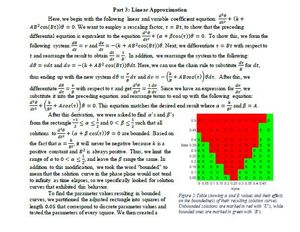CAS MA 226:  Differential Equations
Vertically Forced Pendulum Analysis, Apr. 2015

Studied the equation of motion for a pendulum with a moving pivot point with different damping constants and initial conditions.  Familiarized myself with the long-term behavior of solutions to certain forced second-order equations.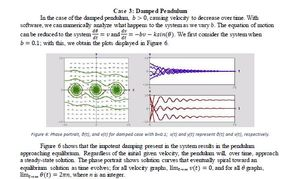CAS MA 226:  Differential Equations
Pendulum Analysis, Mar. 2015

Studied the equation of motion for a pendulum with different damping constants and initial values.  Learned how to analyze and physically interpret the behavior of differential equations with different initial conditions.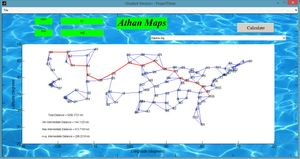ENG EK 127: Introduction to Engineering Computation
Project 3: Shortest Path Algorithm in MATLAB, Dec. 2014

Successfully implemented Dijikstra’s Shortest Path Algorithm in a GUI to find optimal routes between two cities in the United States.  Used a large data file that had latitude and longitude coordinates of various US cities; I imported them into MATLAB and manipulated the data for simplified analysis.  Learned about GUI’s and shortest path algorithms.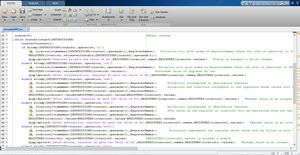ENG EK 127: Introduction to Engineering Computation
Project 2: MIPS Assembly Language in MATLAB, Nov. 2014

Created a MATLAB program that successfully and efficiently reads and executes a MIPS program stored in a text file. Learned about the MIPS assembly language, memory allocation, and CPU processes.

ENG EK 127: Introduction to Engineering Computation
Project 1: Deflection of Cantilever Beams, Oct. 2014

Designed a MATLAB program that determines appropriate I-beam designations for use in a specific design scenario given a set of parameters. Analyzed Cantilever Beam deflections with different applied forces. Learned about integral Mechanical Engineering principles.